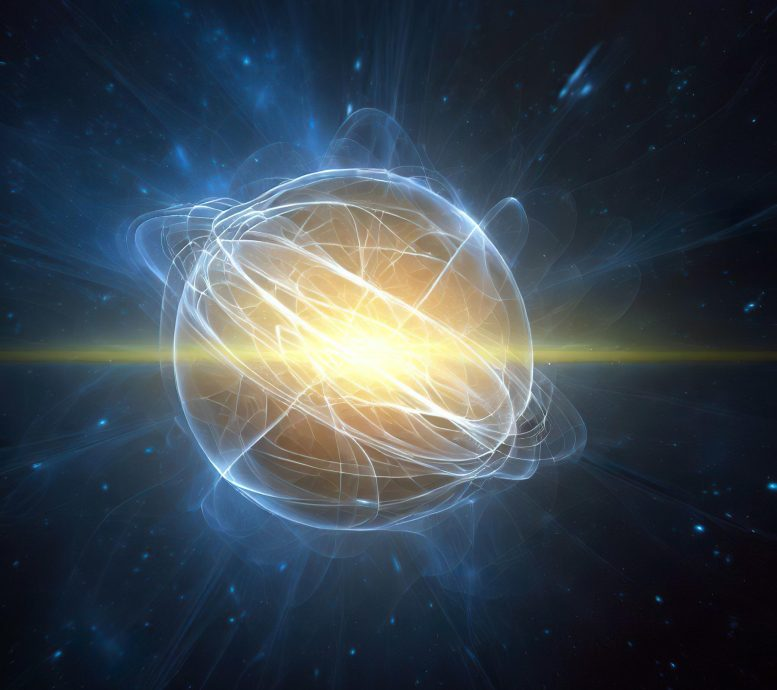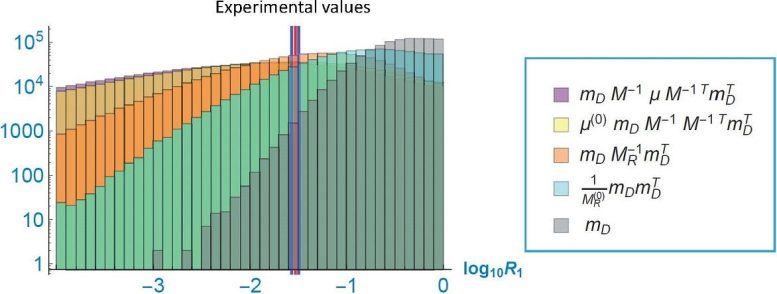# Deciphering the Mystery of Neutrino Mass – A New Approach Uses Random Matrix TheoryScientists have used random matrix theory to theoretically show that neutrino mass hierarchy can be mathematically explained.

When any substance is fragmented into increasingly smaller segments, ultimately, you reach a point where division is no longer possible. At this point, you’re left with an elementary particle. As of now, 12 distinct elementary particles have been identified, composed of a blend of quarks and leptons, each available in six unique variations.

These variants are categorized into three generations. Each generation contains a lepton that carries a charge and one that is neutral, forming various particles such as electron, muon, and tau neutrinos. In the Standard Model, the masses of the three generations of neutrinos are represented by a three-by-three matrix.

A research team led by Professor Naoyuki Haba from the Osaka Metropolitan University Graduate School of Science, analyzed the collection of leptons that make up the neutrino mass matrix. Neutrinos are known to have less difference in mass between generations than other elementary particles, so the research team considered that neutrinos are roughly equal in mass between generations. They analyzed the neutrino mass matrix by randomly assigning each element of the matrix. They showed theoretically, using the random mass matrix model that the lepton flavor mixings are large.The horizontal axis shows the ordinary logarithm of the neutrino mass squared difference ratio, while the vertical axis shows their probability distribution. Each histogram represent the probability distributions for the seesaw mechanisms of the corresponding color. The vertical red and blue lines represent the experimental values (1σ and 3σ errors) of the ordinary logarithm of the neutrino mass squared difference ratio. The probability distribution for the seesaw model with the random Dirac and Majorana matrices in orange has the highest probability of reproducing the experimental value. Credit: Naoyuki Haba, Osaka Metropolitan University

“Clarifying the properties of elementary particles leads to the exploration of the universe and ultimately to the grand theme of where we came from!” Professor Haba explained. “Beyond the remaining mysteries of the Standard Model, there is a whole new world of physics.”

After studying the neutrino mass anarchy in the Dirac neutrino, seesaw, double seesaw models, the researchers found that the anarchy approach requires that the measure of the matrix should obey the Gaussian distribution. Having considered several models of light neutrino mass where the matrix is composed of the product of several random matrices, the research team was able to prove, as best they could at this stage, why the calculation of the squared difference of the neutrino masses are closest with the experimental results in the case of the seesaw model with the random Dirac and Majorana matrices.

“In this study, we showed that the neutrino mass hierarchy can be mathematically explained using random matrix theory. However, this proof is not mathematically complete and is expected to be rigorously proven as random matrix theory continues to develop,” said Professor Haba. “In the future, we will continue with our challenge of elucidating the three-generation copy structure of elementary particles, the essential nature of which is still completely unknown both theoretically and experimentally.”

Reference: “Neutrino mass square ratio and neutrinoless double-beta decay in random neutrino mass matrices ” by Naoyuki Haba, Yasuhiro Shimizu and Toshifumi Yamada, 19 January 2023, Progress of Theoretical and Experimental Physics.

The study was funded by the Ministry of Education, Culture, Sports, Science and Technology of Japan.

#### 3 Commentson "Deciphering the Mystery of Neutrino Mass – A New Approach Uses Random Matrix Theory"

1.Bao-hua ZHANG | July 4, 2023 at 6:06 pm | Reply

When any substance is fragmented into increasingly smaller segments, ultimately, you reach a point where division is no longer possible. At this point, you’re left with an elementary particle. As of now, 12 distinct elementary particles have been identified, composed of a blend of quarks and leptons, each available in six unique variations.
This paragraph is contradictory and full of loopholes. Please tell the public what is the basis for the 12 distinct elementary particles you described where division is no longer possible. According to the topological vortex gravitational field theory, this speech may be suspected of Pseudoscience.

•Bao-hua ZHANG | July 5, 2023 at 11:40 pm | Reply

How to explain that the elementary particle is composed of a blend of quarks and leptons, and where division is no longer possible.

•Bao-hua ZHANG | July 6, 2023 at 1:44 am | Reply

The physical phenomena observed in scientific research are often not comprehensive, let alone essential.